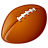# converting 32 bit to 8 bit in python

1944
0
08-15-2011 12:27 PMNew Contributor II
Trying to put together a python script for converting my 5-band, 32 bit unsigned to image to a 3-band, 8 bit unsigned image. I'm doing this in conjunction with calculating an NDVI for each image as well. I start by converting the geo-tif to a ESRI GRID. The next part is what is breaking down for me (highlighted in dark red). I take the 3 raster bands (ESRI GRIDS) that I want to composite and convert them to 8 bit tif files. I then composite them in the order I want into a single. The problem that I am having is that each CopyRaster_management routine I run to convert the bands to 8 bit tif's produces a 8 bit file with all the pixels having the same value. I checked the GRID files and they are correct. Problem is happening in the coversion to 8 bit. NDVI routine runs fine as it continues to work fro the GRID files. Anybody see something that I'm missing?

`import arcpyimport osfrom arcpy import safrom arcpy.sa import *arcpy.env.overwriteOutput = True arcpy.CheckOutExtension("spatial") inDIR = "C:/GIS_Projects/5_band/clipped"bitDIR = "C:/GIS_Projects//images/bit_8"ndviDIR = "C:/GIS_Projects/images/ndvi"tmpDIR = "C:/Temp/grids"arcpy.env.workspace = inDIRrasters = arcpy.ListRasters("*", "TIF")for raster in rasters: arcpy.env.workspace = inDIR print raster fltname = raster[:-9] rname = raster[:-9] + ".tif" ndviname = raster[:-9] + "_ndvi.tif" temp = "temp" arcpy.CopyRaster_management(raster, os.path.join(tmpDIR,temp), "", "", "", "NONE", "NONE", "32_BIT_UNSIGNED") print "Converting " + rname + " to 8 Bit Image" arcpy.env.workspace = tmpDIR arcpy.CopyRaster_management("tempc5", "band5.tif", "", "", "", "NONE", "ColormapToRGB", "8_BIT_UNSIGNED") arcpy.CopyRaster_management("tempc3", "band3.tif", "", "", "", "NONE", "ColormapToRGB", "8_BIT_UNSIGNED") arcpy.CopyRaster_management("tempc2", "band2.tif", "", "", "", "NONE", "ColormapToRGB", "8_BIT_UNSIGNED") arcpy.CompositeBands_management("band5.tif;band3.tif;band2.tif", os.path.join(bitDIR,rname))print "Converting " + rname + " to float" arcpy.env.workspace = inDIR arcpy.RasterToFloat_conversion(os.path.join(tmpDIR,temp + "c3"), os.path.join(tmpDIR, fltname + "_c3.flt")) arcpy.RasterToFloat_conversion(os.path.join(tmpDIR,temp + "c5"), os.path.join(tmpDIR, fltname + "_c5.flt")) arcpy.CopyRaster_management(os.path.join(tmpDIR, fltname + "_c5.flt"), os.path.join(tmpDIR, \ "nir.tif"), "", "", "", "NONE", "NONE", "32_BIT_FLOAT")  arcpy.CopyRaster_management(os.path.join(tmpDIR, fltname + "_c3.flt"), os.path.join(tmpDIR, \ "red.tif"), "", "", "", "NONE", "NONE", "32_BIT_FLOAT") print "Calculating NDVI for " + rname divide = arcpy.sa.Divide(arcpy.sa.Minus(tmpDIR + "/" + "nir.tif", \ tmpDIR + "/" + "red.tif"), arcpy.sa.Plus(tmpDIR + "/" + "nir.tif", tmpDIR + "/" + "red.tif"))  divide.save(os.path.join(ndviDIR, ndviname))`
Tags (3)
0 Replies